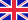•# Pythagorean Tuning

Autor: Lambert M. Surhone

Pythagorean tuning is based on a stack of perfect fifths, each tuned in the ratio 3:2, the next simplest ratio after 2:1, which is the ratio of an octave. Starting from D for example, the A is tuned such that the frequency ratio of A and D is 3:2 - if D... Viac o knihe

Na objednávku, dodanie 2-4 týždne

25.42 €

bežná cena: 29.90 €

## O knihe

Pythagorean tuning is based on a stack of perfect fifths, each tuned in the ratio 3:2, the next simplest ratio after 2:1, which is the ratio of an octave. Starting from D for example, the A is tuned such that the frequency ratio of A and D is 3:2 - if D is tuned to 288 Hz, then the A is tuned to 432 Hz. The E above A is also tuned in the ratio 3:2 - with the A at 432 Hz, this puts the E at 648 Hz, 9:4 above the original D. When describing tunings, it is usual to speak of all notes as being within an octave of each other, and as this E is over an octave above the original D, it is usual to halve its frequency to move it down an octave. Therefore, the E is tuned to 324 Hz, a 9:8 above the D. The B at 3:2 above that E is tuned to the ratio 27:16 and so on. Starting from the same point working the other way, also from D to G is tuned as 3:2. With D at 288 Hz, this arrives at G at 192 Hz, or, brought into the same octave, to 384 Hz.

• Vydavateľstvo: Betascript Publishers
• Rok vydania: 2009
• Formát: Paperback
• Rozmer: 220 x 150 mm
• Jazyk: Anglický jazyk
• ISBN: 9786130309619

Generuje redakčný systém BUXUS CMS spoločnosti ui42.